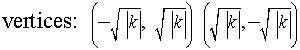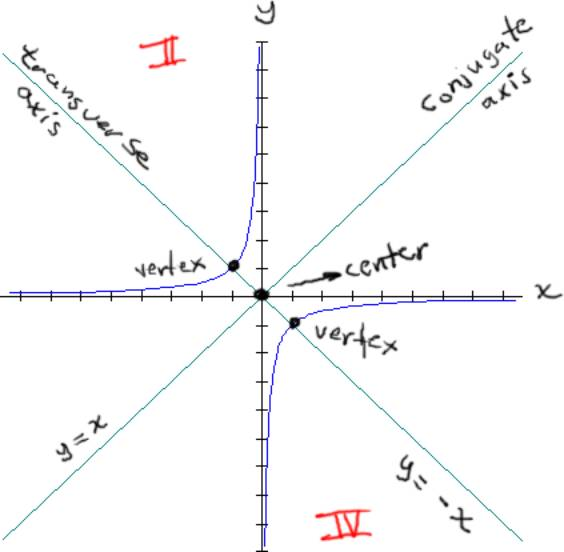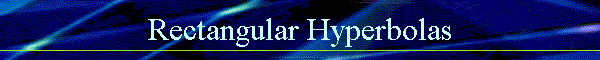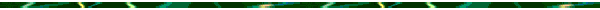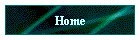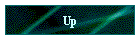Rectangular Hyperbolas   Rectangular Hyperbolas (or Equilateral Hyperbolas) are hyperbolas in the form of:  xy = k, where k ¹ 0.   If k = a positive number, then  1) The branches of the hyperbola lie in Quadrant I and III  2) The center is (0, 0)  3) The asymptotes are the x- axis and the y-axis.  4) The Transverse axis of symmetry is: y = x  5) The Conjugate axis of symmetry is: y = -x  6) The Domain is the set of all real numbers, except x   0.  7) The vertices are located where the transverse axis intersects the graph of the hyperbola.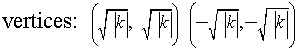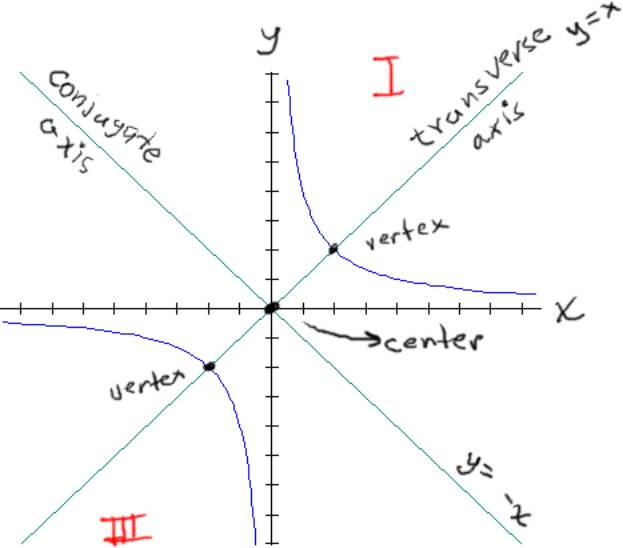If k = a negative number, then  1) The branches of the hyperbola lie in Quadrant II and IV  2) The center is (0, 0)  3) The asymptotes are the x- axis and the y-axis.  4) The Transverse axis of symmetry is: y = -x  5) The Conjugate axis of symmetry is: y = x  6) The Domain is the set of all real numbers, except x   0.  7) The vertices are located where the transverse axis intersects the graph of the hyperbola.